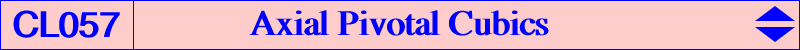Let (L) be a line and let A', B', C' be the reflections of A, B, C about (L). There is a unique circumconic (C) passing through A', B', C'. Let T be the trilinear pole of (L) and let Z be the perspector of (C). The isoconjugation f that swaps T and Z transforms (L) into (C) and vice versa. Let Ω be the pole of f i.e. the barycentric product of T and Z. In the sequel, we shall write M* = f(M). Let X be the infinite point of (L) and Y that of any perpendicular to (L). The reflection of Y* about (L) is P. Note that P is also the reflection of T about Y*. Theorem : pK(Ω, P) = pK(L) is an axial pivotal cubic invariant under the symmetry with axis (L). Note that pK(L) meets the line (L) at three real points A", B", C" which are the isoconjugates of A', B', C'. pK(L) has always one real asymptote, the perpendicular to (L) at Z. The two other asymptotes are not necessarily real. *** When (L) passes through O, the conic (C) is the circumcircle. In this case, • Ω lies on the circumconic with perspector X(184) passing through X(112), X(248), X(906), X(1415), X(1576), X(2966), • the real asymptote passes through K, • the two other asymptotes are real if and only if ABC is obtusangle, • the orthic line passes through X(184). • the isogonal transform of pK(L) is a central pK with pole on the orthic axis, pivot on C(X4, 2R), center on the nine points circle. See K528 (axial cubic) and K255 (central cubic) for instance. See also K335 (axial cubic). *** Two other axial pK(L) are presented in CL056 when (L) is the orthic axis and the antiorthic axis.